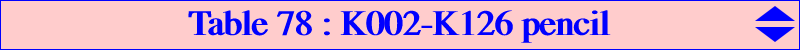The Thomson cubic K002 and K126 are two K0 circum-cubics passing through H and K, hence they generate a pencil of cubics of the same type. Their four remaining common points consist of two pairs of points {M1,M2}, {N1,N2} that lie on the axes of the orthic inconic (center K, perspector H), or equivalently, the parallels at K to the asymptotes of the Jerabek hyperbola. The points in each pair are G-Ceva conjugate since they are collinear with the isopivot K of K002. More precisely, • M1, M2 lie in the line {6, 1344, 2574} and on the rectangular circum-hyperbola passing through X(1113). Their midpoint is X(1344) and their barycentric product is X(25). • N1, N2 lie in the line {6, 1345, 2575} and on the rectangular circum-hyperbola passing through X(1114). Their midpoint is X(1345) and their barycentric product is also X(25). *** This pencil contains several other remarkable cubics, namely : • K002 and two other pKs : K445 = pK(X2207, X4) and pK(X25, X6). • three nK0s : nK0(X25, X523) and the others are decomposed into one axis of the orthic inconic and a rectangular circum-hyperbola passing through X(1113) or X(1114) and the points of the pair on the other axis. • K1251 which is the orthopivotal cubic O(X1995), a circular cubic with singular focus X(11579) passing through X4, X6, X13, X14, X30, X111, X1995, X5523, X34288, X34802. • K643, a stelloid with radial center X(11579) passing through the infinite points of K003, the vertices of the Grebe triangle and X(i) for these i : {4846, 8743, 39641, 39642}. • two other K+ (apart K643) with asymptotes concurring at G and X(1597) respectively. *** Every cubic of the pencil meets the line at infinity and the circumcircle at the same points as two isogonal pKs with pivots P1 on the Euler line and P2 on the line GK respectively. Moreover, the line P1P2 is parallel to the Brocard axis.